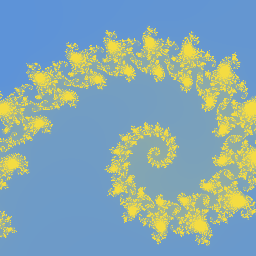# CPSC170AFundamentals of Computer Science II

## Project 1

MandelbrotThe Mandelbrot set is a set of complex numbers that when graphed on the complex plane has a boundary with a complex pattern that does not simplify at any magnification.

A complex number, $$c_0 = a+bi$$, is in the Mandelbrot set if the absolute value of the complex numbers computed with the recursive quadratic polynomial function remain bounded. The recursive quadratic polynomial function is defined as:

$$c_n = c_{n-1}^2 + c_0$$

Two complex numbers are added using the equation:

$$(a_1 + b_1i) + (a_2 + b_2i) = (a_1 + a_2) + (b_1 + b_2)i$$

Two complex numbers are multiplied using the equation:

$$(a_1 + b_1i)(a_2 + b_2i) = (a_1a_2 - b_1b_2) + (b_1a_2 + a_1b_2)i$$

The absolute value of a complex number is computed using the equation:

$$\lvert a + bi \rvert = \sqrt{a^2+b^2}$$

#### Details

Create a C++ program that prints a ppm file that visualizes a region of Mandelbrot set. Produce a visualization of the Mandelbrot set by converting the coordinates of every pixel in the image to a complex number and converting every complex number into a color using the quadratic polynomial function.

Convert a pixel’s coordinates to a complex number by using interpolation. That is, if the row of a pixel is located at 25% of the height of the image from the top, then it should have a value for $$b$$ that is 25% of the height from the top of the region of the complex plane that is being visualized. The program should visualize a region of the complex plan that is interesting. The above image is centered on the point $$0 + 0i$$, with a width and height of 4 and shows the entirety of the Mandelbrot set. More interesting regions can be found here. The center and extent of the region being visualized should be stored in global constant variables.

Convert a complex number to a color by first finding the complex number’s escape depth. The escape depth is the number of calls to the recursive quadratic polynomial function before the absolute value of computed complex number exceeds the bound of $$2.0$$. Note that many complex numbers do not exceed the the bound. To prevent infinite recursion, the computed escape depth should be limited to a fixed maximum. The maximum escape depth should be stored in a global constant variable. Again, use interpolation to convert the escape depth to a color. That is, if the escape depth is 75% of the maximum escape depth, then the color should be a blend of two colors where it is 75% of one color and 25% of another color. To interpolate two colors, interpolate each of the RGB values that make up the two colors. The RGB values of the two colors used in the interpolation should be stored in global constant variables.

### Extra Credit

Create a video of a zoom into a point in the Mandelbrot set like the one we watched in class. Begin by picking a neat location deep in the Mandelbrot set. And repeatedly render the Mandelbrot set and zoom. Zoom by interpolating between the zoomed in view and a view of the entire set (x=0, y=0, r=4). To interpolate between the views, interpolate between each of the components of the view. That is interpolate the center x coordinate between the two views, interpolate between the center y coordinate of the two views, and interpolate between the range of the two views. Once you have a collection of images, each at a different amount of zoom, use a program like FFmpeg to combine them into a video.

### Collaboration

You may collaborate with a partner on this assignment. If you do, both partners must work on the same computer and actively contribute to every line of code. Only one partner needs to submit code, but both partners names should be in the code.

### Grading

The project will be graded according to the following weights:

10% - Style, design, and documentation

10% - Test cases (and assertions)

30% - Recursively compute escape distance using quadratic polynomial bound function

20% - Convert pixel to complex number in an interesting region

20% - Convert escape distance to color by interpolating between two colors

10% - Print ppm image that visualizes the Mandelbrot set

### Submission

Submit a cpp file containing the function compute_escape_depth(double a0, double b0, double max_depth) and code that tests the function on the course Inquire page before class on Friday February 9th.

Submit a cpp file containing your final program and some cool images you created using it on the course Inquire page before class on Wednesday February 14th.